Follow

# Add questions to an exam

In this guide, we go through the different question types, what the differences are and how they are used.

## Question types

Hover the mouse over the Add question-button. Select the question type you would like to add (figure 1).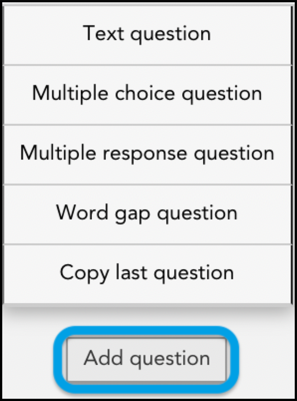Figure 1

## There are four different question types:

Text question:

Students answer with a written answer. A text question is appropriate for open-ended, short answers, fill-ins, and essay questions. The answer can include text, numbers, and drawings (on most operating systems). When grading text questions, it’s possible to comment and provide feedback directly in the student’s answer.

Multiple choice question:

Students are presented with multiple answer choices and select only one answer choice as correct. This question type is always corrected automatically.

Multiple response question:

Students are presented with multiple answer choices and select all answer choices that apply. This question type is only corrected automatically if the student gets the answer completely correct or completely incorrect. If the student gets the answer partially correct, it is up to the teacher to go in and manually enter the score.
Word gap question:

This question type means that a text is presented followed by a text box in which the student fills in his/her answer. The answer is then compared to the correct word for a match. "Word Gap" can be used for glossaries, quizzes, questioning, spelling and much more.

## Text question

Free text questions have no preselected options to choose from.

A free text question can consist of all the usual characters and with the possibility of limiting the number of words (see soft and hard limitation) that the student can write.

The teacher himself puts points on free text questions and can also give feedback to the student in different ways (described in more detail under "correction and feedback") during the correction. (Figure 2)

Fill in the following fields as required (Figure 2):

Question title Edit the question title/number as needed.

Question: Enter your question in the text box. This is a required field.

Max points: The number of possible points students can earn for the question. This is a required field.

Max length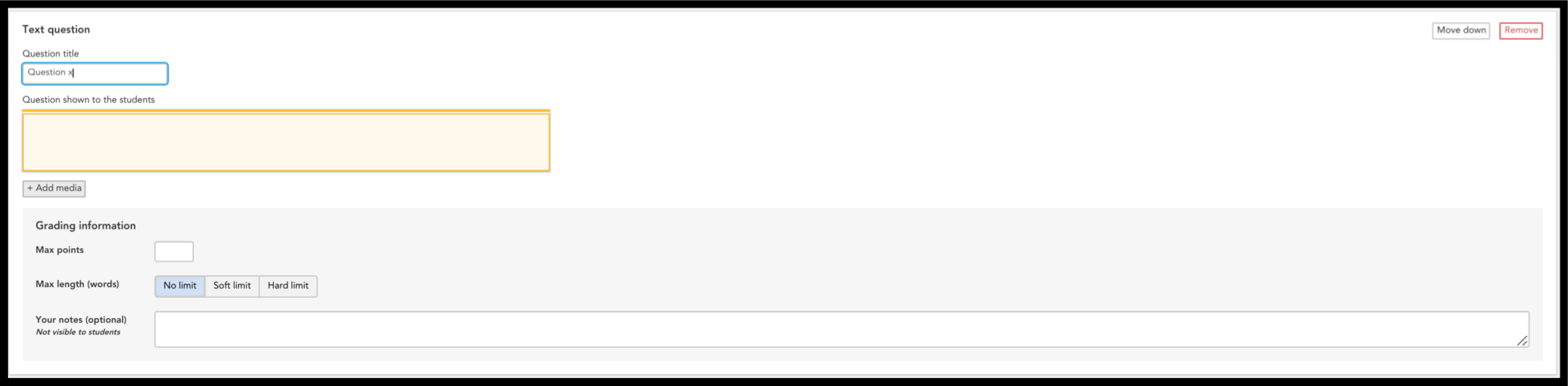Figure 2

## Multiple choice question

Multiple choice question with two or more options, only one option can be correct (see image below).
(* Please note that once the student selects an answer using this type of question, they cannot deselect their answer, only change it to a different one.)

List two or more answer choices/alternatives.

Use the remove and add alternatives buttons until you have the number of answer choices you need.

Only one answer choice/alternative can be correct.  Select which answer choice is correct.

Max points: The number of possible points students can earn for the question.  This is a required field.

Your notes: Notes are not visible to students. Professors and teachers might use notes to list what should be included in a student answer, or to provide background information on a question when sharing exams with colleagues, etc.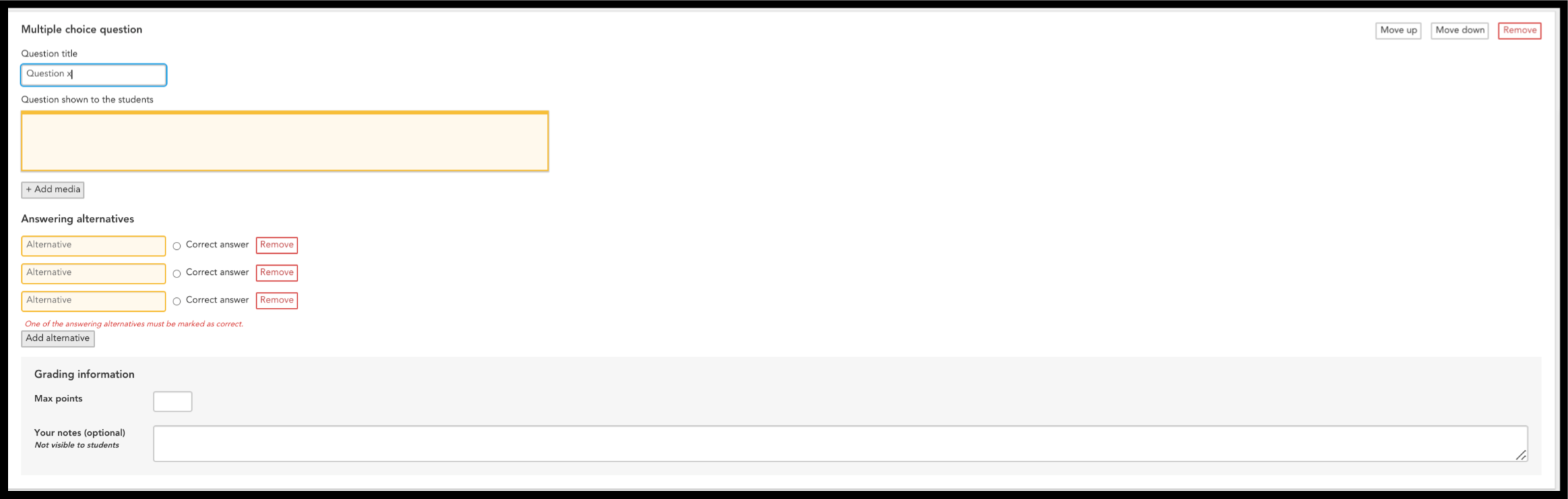Figure 3

## Multiple response question

Fill in the following fields to meet your needs (figure 4):

Use the remove and add alternatives buttons until you have the number of answer choices you need. Many of the answer choices/alternatives can be correct.  Select which answer choices are correct.

All or nothing: The student must complete all the correct spellings to get points. If the student makes a misspelling or writes an incorrect word, the student is assigned zero points.

Right minus wrong: Students receive plus points for correct spelling and minus points for each incorrect spelling.

Points per answer: Each correct spelling gives plus points and if the spelling of a gap is wrong it won't give any points. Each correct answer is worth the maximum points divided by the number of gaps.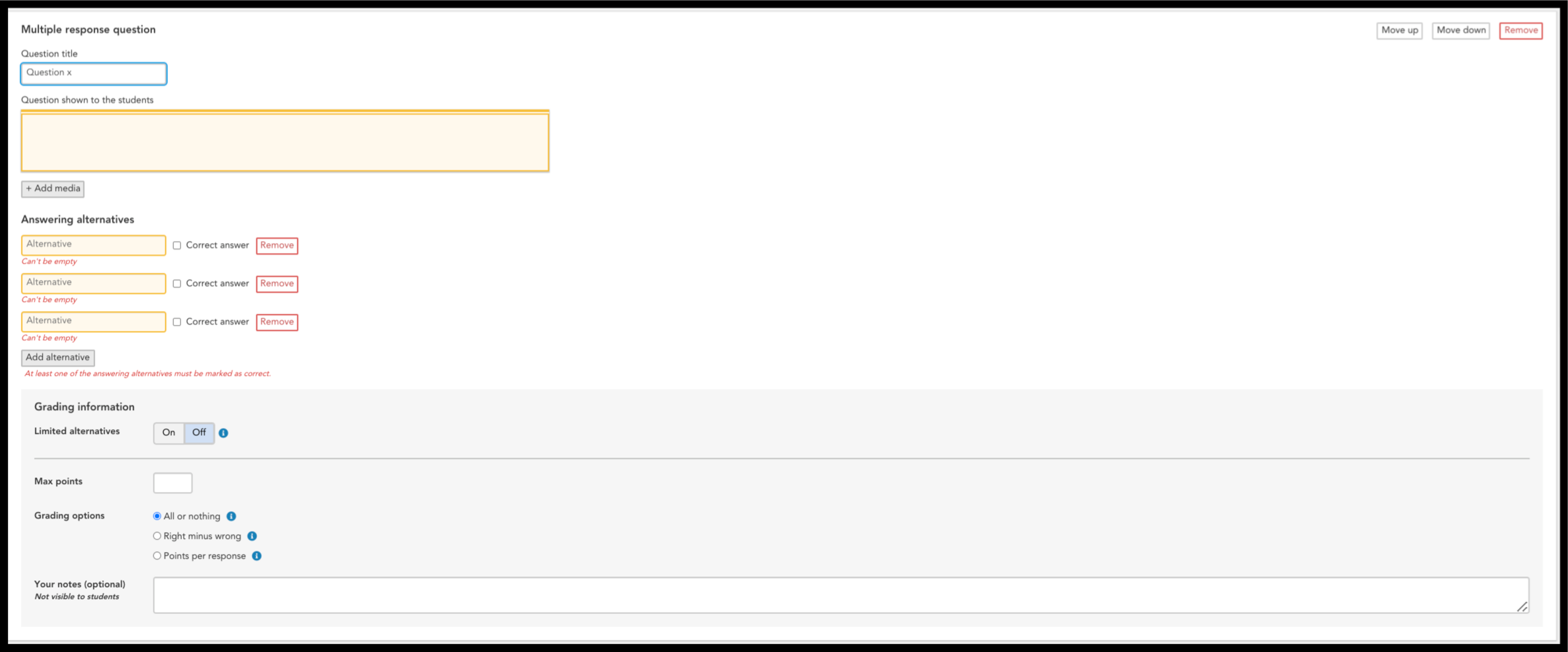Figure 4

## Word gap question

NOTE! Word gap only works on the Digiexam client with version 11.4.0 and later.

This question type means that a text is presented followed by a text box where the student fills in their answer. The response is then compared to a list of allowed responses. Word gaps can be used for vocabulary, quizzes, interrogations, spelling and much more. (Figure 5)

Fill in the following fields to meet your needs (figure 5):

Matching types:

Exact Match: This option requires that the blank is filled in exactly as the teacher wrote.

Contains: With this setting, it does not need to be exactly that word but the word must be included.

Case-sensitive: makes the words sensitive to upper and lower case letters.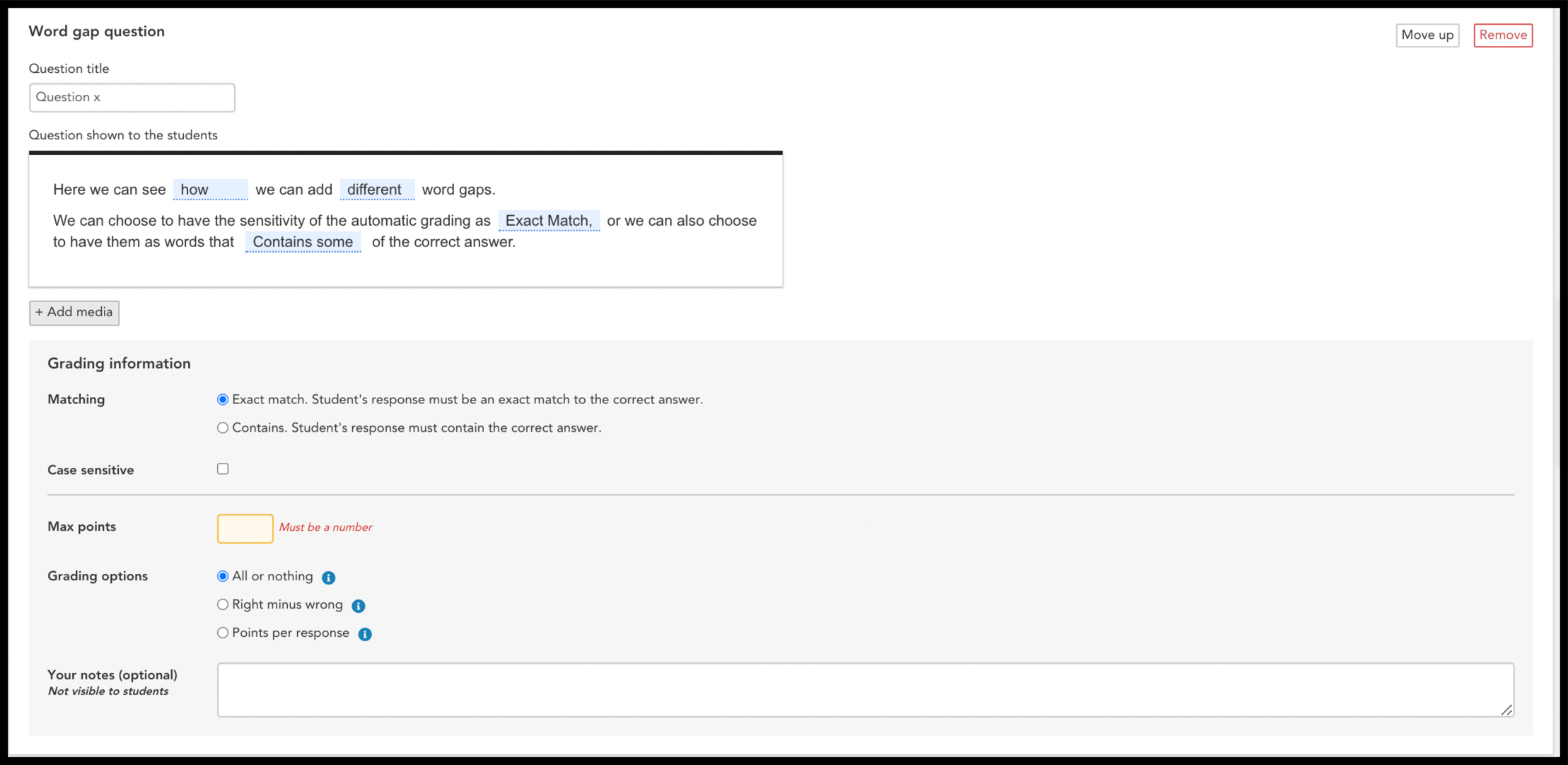Figure 5

### Grading options for multiple choice question and word gaps:

All or nothing: The student must complete all the correct spellings to get points. If the student makes a misspelling or writes an incorrect word, the student is assigned zero points.

Right minus wrong: Students receive plus points for correct spelling and minus points for each incorrect spelling.

Points per answer: Each correct spelling gives plus points, and if the spelling of a gap is wrong it won't give any points. Each correct answer is worth the maximum points divided by the number of gaps.

### Max length (words) for text questions:

No limit allows students to write any number of words.

Soft limit Students are able to continue adding text to their answer even after they’ve reached the maximum word limit. The word counter will turn red to let them know they have exceeded the quota.

Hard limit  The student is unable to write more than the specified word limit.Figure 6

If you want to insert an image, you can do so by clicking on Insert image in the text editor.

You can attach one or more images. Digiexam supports JPEG, GIF, PNG and TIFF files.

NOTE! Only images work offline, all other media links require an internet connection to work.

You can also add your own notes that are not visible to the students to each question. You will then see these notes when grading the test (Figure 7).(Figure 7)

## Save the exam

Select the green Save-button and the exam will appear in your library (figure 8).  If the exam does not appear in your library, then it means one of the mandatory fields has been left blank.  Click on the red box with a question mark to see what is missing.  Add in any missing information and try to save your exam again.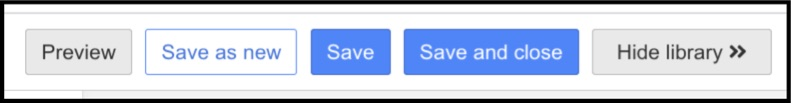Figure 8

Next article: Start an exam